# Demonstrating that the Angles in a Triangle Sum up to 180 Degrees

We are taught that the sum of the angles in a triangle add up to 180°

Here is a simple way to demonstrate that fact.

A proof of the angles of a triangle summing to 180° can be found here.

### Materials

• paper
• pencil or pen
• ruler (or some sort of straight-edge)
• coloured crayon
• scissors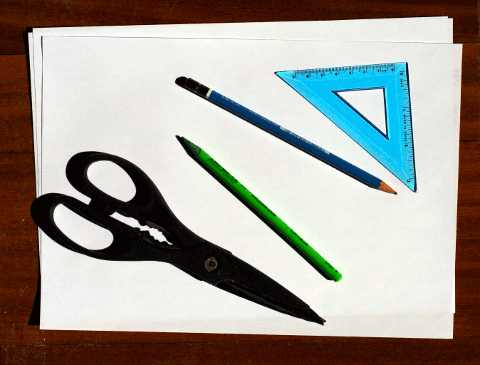1) Draw a triangle on a sheet of paper. Any sort of triangle you like. Use the ruler or straight-edge to ensure the sides are straight: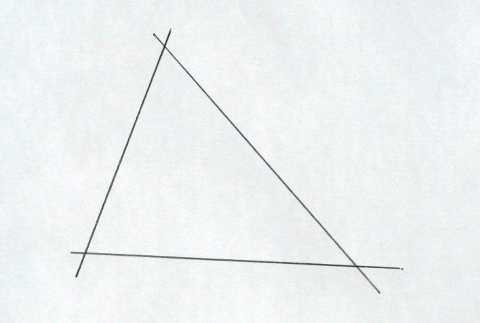2) Colour the edges of the triangles. Do not colour the inside of the triangle. If you really want to colour the inside of the triangle, then use a different colour for the inside from the edges: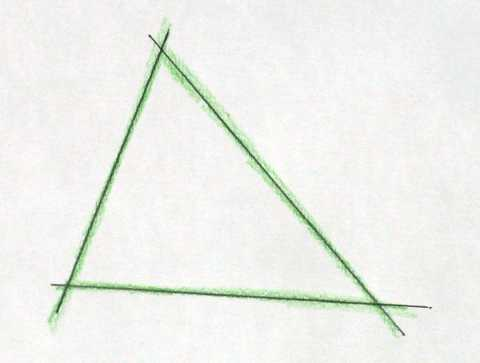3) Cut out the triangle. Make sure the edges are as straight as possible: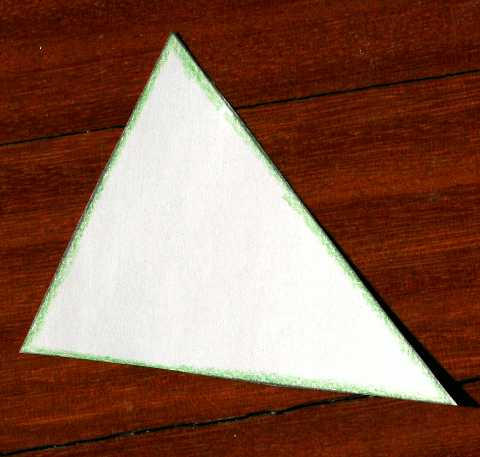4) Cut the corners off the triangle. Make the corners large enough so they are easy to handle: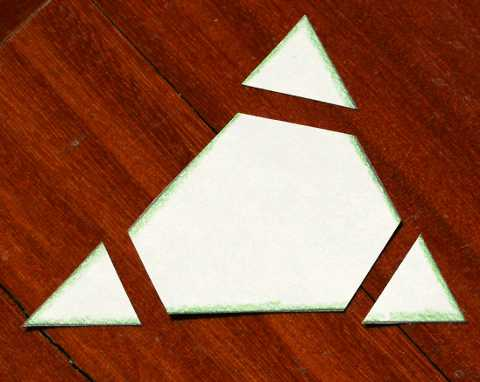Notice that each corner has two coloured edges and one uncoloured edge. (I should have used a more contrasting colour than green – maybe red – or else pressed harder when I coloured.

5) Draw a straight line on a sheet of paper using the ruler (I used one of the scraps from the paper I cut the triangle out of):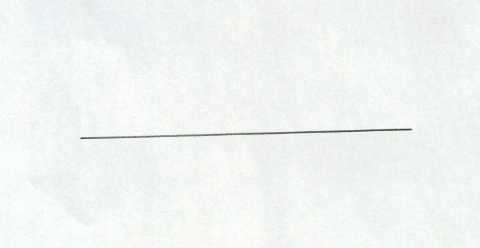6)Assemble the corners on the straight line. Ensure that (1)coloured edges touch the straight line and (2) coloured edges touch other coloured edges: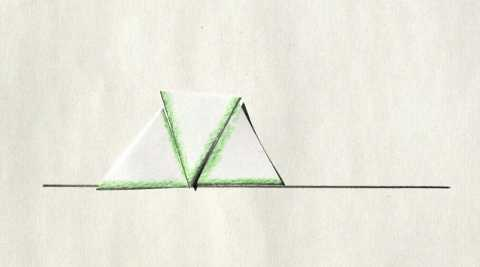## What’s Happening

Each corner that you cut off contains an angle from the triangle. This is why we coloured the edges so we can easily see the angle contained by the edges.

When we assemble the angles (by aligning the coloured edges), we see that all the angles add up to a straight line (or 180°). In other words: Angle 1 + Angle 2 + Angle 3 = 180°